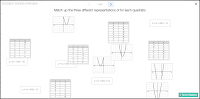## Thursday, 12 March 2015

### Sort students into groups using Quadratic RepresentationsIn this activity students are each given one card. The card will either have a graph, table of values or equation of a quadratic relation. Their job is to find the two other students who have the other two representations of the same quadratic relationship. This shouldn't take too long and could be repeated every couple of days to solidify conversion between representations.
New: Alternatively, you could have students just work individually on this Desmos card sort

• MPM2D - A3.3 determine, through investigation, and describe the connection between the factors of a quadratic expression and the x-intercepts (i.e., the zeros) of the graph of the corresponding quadratic relation, expressed in the form y = a(x – r)(x – s);
• MBF3C - A1.8 determine, through investigation, and describe the connection between the factors of a quadratic expression and the x-intercepts of the graph of the corresponding quadratic relation
• MCF3M - A1.5 determine, through investigation, and describe the connection between the factors used in solving a quadratic equation and the x-intercepts of the graph of the corresponding quadratic relation
• MCR3U - As review
• Download the cards and cut them out (you may want to laminate them)
• If you are doing the Desmos Cardsort instead, students should have devices to do the sort on (note that phones have screens that are too small)1. Shuffle the cards and distribute one per student. Note that there are 11 sets of 3 cards so you may want to remove sets to more closely match your student population.
2. Instruct students to find the two other people that have different representations for the same quadratic relation.
3. Once students find their partners they will be in groups of threeIf doing the Desmos Cardsort instead, have students (or pairs of students) complete each page of the cardsort. You may wish to consolidate on the last page of the card sort.
Did you use this activity? Do you have a way to make it better? If so tell us in the comment section. Thanks